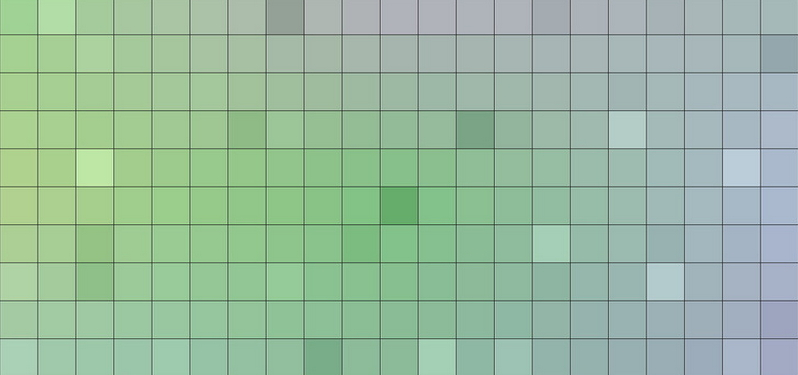# 3dsmax maxscript教程-间隔取一个多边形面小脚本

–建立空数组

n=#()

–分列数。就是一个网格格子纵向的数量

k=32

–数组n的系列排序，初始量为1

u=1

–for循环 a的值等于网格的行数量

for a =0 to 20 do

(

–mod是取余数的运算，当余数等于0时运算代码，不等于0时运行代码

if (mod a 2)==0 then

(

–在if语句中嵌套for循环函数，当等于0时，做k值范围内的循环运算,b的值每次加2取值

for  b=1 to k by 2 do

(

–数组赋值

n[u]=a*k+b

u+=1

)

)

else

(

–同上

for  b=2 to k by 2 do

(

n[u]=a*k+b

u+=1

)

)

)

–定义变量m，把n数组的数据类型转化为bitarray

m=n as bitarray

–选择bitarray值的曲面

\$.EditablePoly.SetSelection #Face m